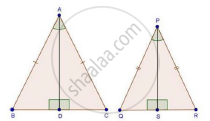Share

Books Shortlist
Your shortlist is empty

# Solution for Two Isosceles Triangles Have Equal Vertical Angles and Their Areas Are in the Ratio 36 : 25. Find the Ratio of Their Corresponding Heights. - CBSE Class 10 - Mathematics

#### Question

Two isosceles triangles have equal vertical angles and their areas are in the ratio 36 : 25. Find the ratio of their corresponding heights.

#### SolutionGiven: AB = AC, PQ = PQ and ∠A = ∠P

And, AD and PS are altitudes

And, ("Area"(triangleABC))/("Area"(trianglePQR))=36/25                  .........(i)

To find "AD"/"PS"

Proof: Since, AB = AC and PQ = PR

Then, "AB"/"AC"=1 and "PQ"/"PR"=1

therefore"AB"/"AC"="PQ"/"PR"

rArr"AB"/"PQ"="AC"/"PR"                ........(ii)

In ΔABC and ΔPQR

∠A = ∠P                                        [Given]

"AB"/"PQ"="AC"/"PR"                 [From (2)]

Then, ΔABC ~ ΔPQR                     [By SAS similarity]

therefore("Area"(triangleABC))/("Area"(trianglePQR))="AB"^2/"PQ"^2            .....(iii) [By area of similar triangle theorem]

Compare equation (i) and (iii)

"AB"^2/"PQ"^2=36/25

"AB"/"PQ"=6/5                 ..........(iv)

In ΔABD and ΔPQS

∠B = ∠Q                          [ΔABC ~ ΔPQR]

∠ADB = ∠PSQ                  [Each 90°]

Then, ΔABD ~ ΔPQS         [By AA similarity]

therefore"AB"/"PQ"="AD"/"PS"

rArr6/5="AD"/"PS"              [From (iv)]

Is there an error in this question or solution?

#### Video TutorialsVIEW ALL 

Solution for question: Two Isosceles Triangles Have Equal Vertical Angles and Their Areas Are in the Ratio 36 : 25. Find the Ratio of Their Corresponding Heights. concept: Areas of Similar Triangles. For the course CBSE
S